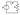# ⚛ Ohm's Law Calculator

## Ohm's Law calculates the relation betwixt Voltage, Current and Resistance. Enter a minimum of 2 values to get the third value.

Resistance (R)

Ohms (Ω)

Current (I)

Amps (A)

Voltage (V)

Volts (V)### Ohm's Law

Ohm's law affirms that the current through a conductor betwixt two points are directly proportional to the voltage crossed the two points.
The voltage in volts (V) is equal to the current (I) in amps (A) times the resistance R in ohms (Ω):
V = I x R
R = V / I
I = V / R